# How to Implement the Weak Form for Time-Dependent Equations

September 27, 2018

In a previous blog series on the weak formulation, my colleague Chien Liu introduced the weak form for stationary problems and the methodology to implement it in the COMSOL Multiphysics® software. This blog post is an extension of the weak form series, showing how to solve a time-dependent problem using the Weak Form PDE interface. As you will see, not much changes in the procedure. Allow us to demonstrate…

### Example Problem: A Transient Partial Differential Equation

Let’s consider the initial boundary value problem given by the 1D time-dependent partial differential

(1)

\frac{\partial{T}}{\partial{t}} = \frac{\partial^2{T}}{\partial{x^2}}, \quad 1 \leq x \leq 5, \quad t \ge 0,

This differential has the boundary conditions

(2)

\frac{\partial{T}}{\partial{x}} = 0 \text{ at } {x = 1}, \quad \forall t

and

(3)

\frac{\partial{T}}{\partial{x}} = -\left({T-1}\right) \text{ at } {x = 5}, \quad \forall t

as well as the initial condition

(4)

T = 3 \text{ at } {t = 0} ,\quad \forall x

This equation has only first-order time derivatives, and as such, we need to specify initial values only for the primary variable T. For an equation with second-order time derivatives, we have to provide initial conditions for the rate \dot{T} as well.

### Obtaining the Weak Form of the Transient Problem

Deriving the weak form for a time-dependent partial differential equation (PDE) follows the same procedure as shown in detail for a stationary problem in Part 1 of the previous blog series. To recap, we first write the PDE as \mathcal{L}(T)=0. In today’s problem, we have

\mathcal{L}(T) = \frac{\partial{T}}{\partial{t}}-\frac{\partial^2{T}}{\partial{x^2}}=0.

Next, enforce \mathcal{L}(T)=0 in a weak sense; that is, integrating the product of this equation and any test function (also known as the weighting function) \tilde{T} over the domain should return 0. Third, apply the product rule of derivatives and the divergence theorem to reduce (weaken) the highest order spatial derivative of the dependent variable T in the weighted integral. In 1D, this boils down to integration by parts, which gives the weak form.

Thus, multiplying (1) by a test function and integrating over the domain, we obtain

(5)

\int_{1}^{5} \tilde{T}\left( \frac{\partial{T}}{\partial{t}}-\frac{\partial^2{T}}{\partial{x^2}} \right) dx = 0, \quad \forall \tilde{T} .

By applying integration by parts on the product with the highest order spatial derivative, we get

\int_{1}^{5} \left(\tilde{T} \frac{\partial{T}}{\partial{t}}-\tilde{T}\frac{\partial^2{T}}{\partial{x^2}} \right) dx=\int_{1}^{5} \left(\tilde{T} \frac{\partial{T}}{\partial{t}}-\left[\frac{\partial}{\partial x}( \tilde{T}\frac{\partial T}{\partial x})-\frac{\partial \tilde{T}}{\partial x} \frac{\partial T}{\partial x} \right]\right) dx = 0 \quad \forall \tilde{T}.

(6)

\Rightarrow \int_{1}^{5} \left(\tilde{T} \frac{\partial{T}}{\partial{t}} +\frac{\partial \tilde{T}}{\partial x} \frac{\partial T}{\partial x} \right) dx-\left[ \tilde{T} \frac{\partial{T}}{\partial{x}} \right]_{x=1}^{x=5}=0\quad\forall \tilde{T}.

To this weak form, we can incorporate the boundary conditions to obtain

(7)

\int_{1}^{5}\left( \tilde{T} \frac{\partial{T}}{\partial{t}} +{\frac{\partial{\tilde{T}}}{\partial{x}} \frac{\partial{T}}{\partial{x}} }\right)dx + \left[ \tilde{T} \left(T-1\right) \right]_{x=5} = 0,\quad \forall \tilde{T}.

Notice that the presence of a time derivative has not changed how we derive the weak form. The partial time derivative just gets multiplied by the weighting function in the above derivation. The integration in the weak form is spatial integration, and as such, we do not do any integration by parts on the time derivative. The weighting function \tilde{T} is a function of spatial coordinates only, whereas the unknown T is a function of both space and time.

### Specifying the Problem in the Weak Form PDE Interface

Specifying a weak form of a transient PDE involves the following three steps in the Weak Form PDE interface in COMSOL Multiphysics:

1. Entering domain contributions
2. Entering boundary conditions
3. Specifying initial conditions

The domain terms are entered in the Weak Expressions field in the Settings window of the Weak Form PDE 1 node. For the problem at hand, this is test(T)*Tt + test(Tx)*Tx, where test(T) represents the weighting (test) function \tilde{T}, which corresponds the dependent variable T. Also, Tt and  Tx are the time and spatial partial time derivatives, respectively, of the dependent variable T.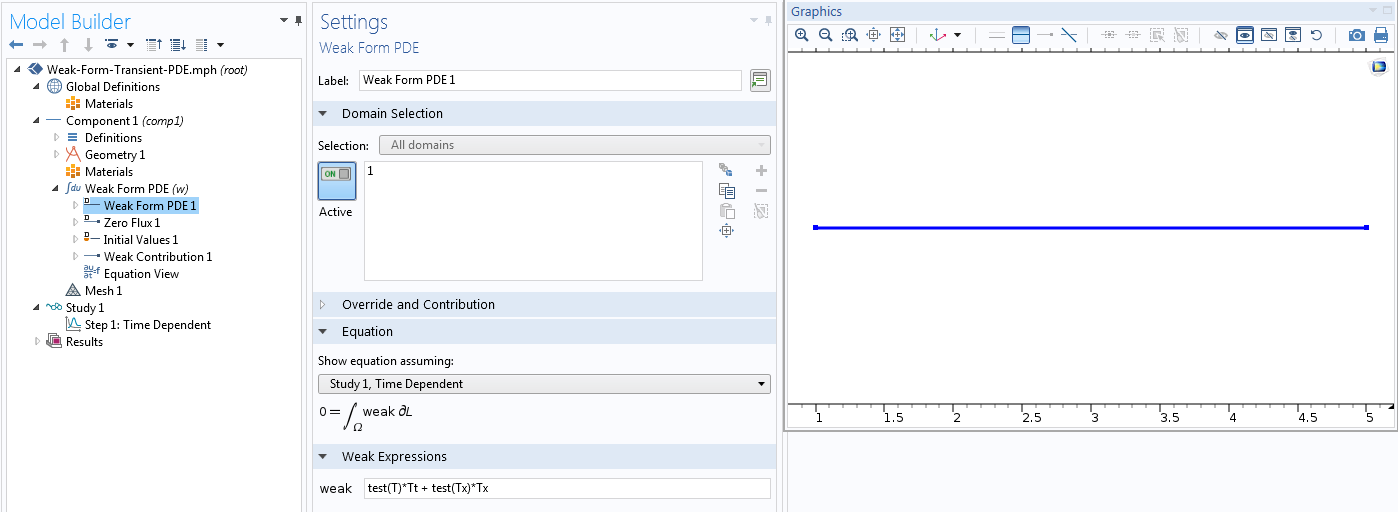Implementation of the domain terms.

The boundary contributions can be specified using one of the built-in boundary conditions or a weak contribution at a boundary. For pedagogic reasons, we will use the latter, even though the boundary conditions in today’s problem could be specified using the built-in Flux/Source node.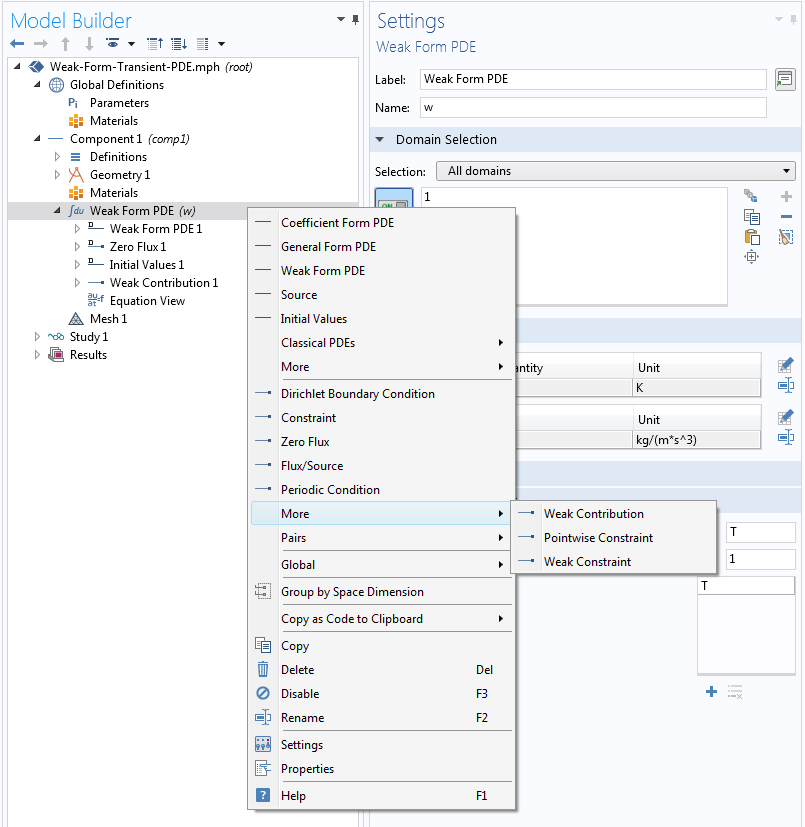Implementation of the boundary terms.

The contribution from the left boundary is zero; we do not have to do anything about that. The contribution from the right boundary, which is the second term in the weak form equation (7), can be entered as follows. We choose point 2 in the Boundary Selection window and enter the expression test(T)*(T-1) in the Weak Expression field.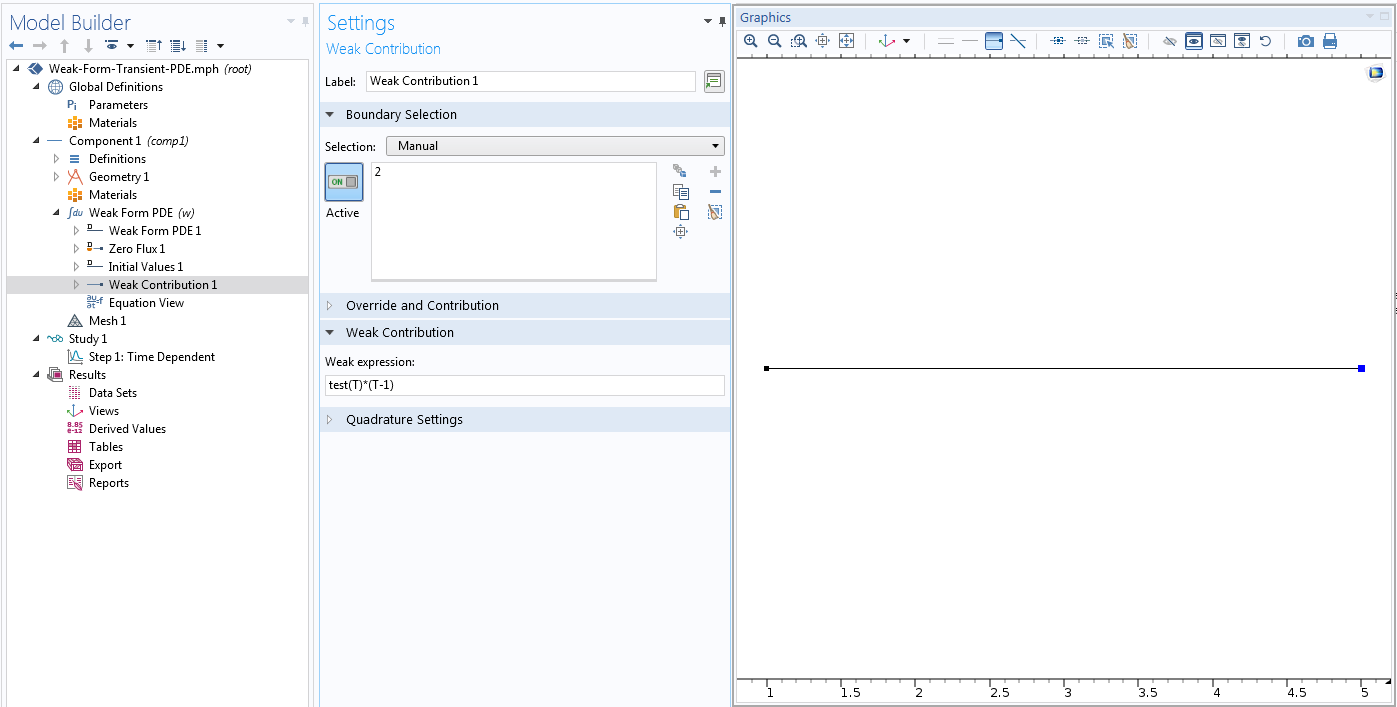Implementation of the boundary terms using the Weak Contribution node.

The last ingredient is the initial value. Our problem needs an initial value on the dependent variable only. Thus, we need to fill out only the first spot in the Settings window for the Initial Values node. In our example, we are considering a uniform initial value. If that is not the case, an expression or function containing spatial coordinates can be typed instead of a number.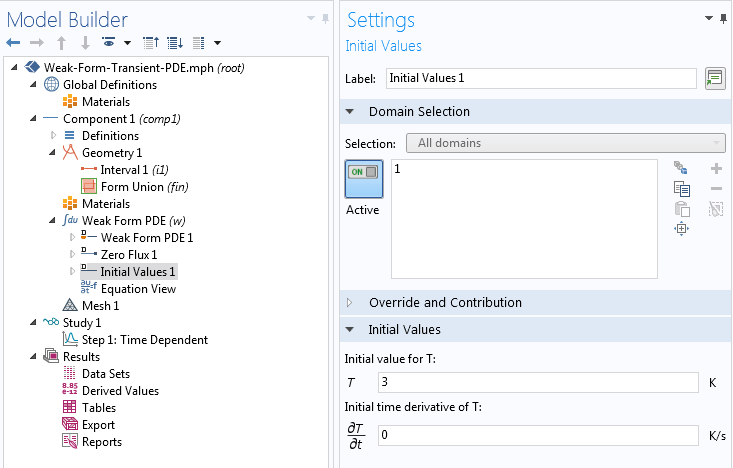Implementation of the initial conditions.

Now that we have specified the PDE, boundary conditions, and initial conditions, all that is left to do in the study settings is to provide the interval of time that we want to solve this problem for and hit the Compute button. The simulation is run for a time range of \left[ 0, 50 \right] s, and the solution at various time instances is shown below.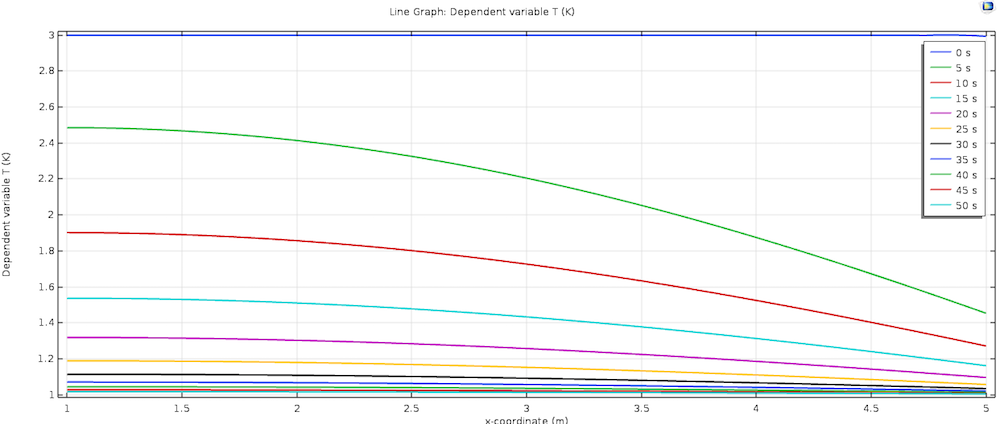Variation of the temperature along the domain at various time instances.

### What Happens Behind the Scenes with the Derived Weak Form

The weak form we derived above and specified in the COMSOL Multiphysics interface is a continuous problem. Internally, this will be discretized using finite element interpolation functions based on the shape function picked in the Discretization section of the Settings window for the Weak Form PDE interface. For the Lagrange interpolation function, for example, the solution is approximated as

T(\mathbf{x},t) = \sum_{i=1}^{N}T_i(t)\phi_i(\mathbf{x}),

where N is the number of nodes in our finite element mesh and \phi_i, i=1\dotsc N defines the shape (interpolation) functions.

If we substitute this in the weak form, we see that we get a system of ordinary differential equations (ODEs) for the nodal solutions T_i(t), i=1\dotsc N. All of the spatial derivatives go to the shape functions, which are known. This system of ODEs is solved by the time integration algorithms built into COMSOL Multiphysics. (Note that we do not need to do any time discretization in our problem specification; that is taken care of internally. This section is just for your information.) If the problem is stationary, the system of equations is an algebraic system for the nodal unknowns T_i. This time, the nodal unknowns are not functions of time. To learn more about discretization, check out this blog post on shape functions and associated discretizations.

### Concluding Thoughts on Obtaining the Weak Form for Transient Problems

In this blog post, we have demonstrated how to solve a transient PDE using the Weak Form PDE interface. The derivation of a weak form for a transient problem follows exactly the same procedure as a stationary problem, and the same interface is used as well. We only need to specify the continuous version of the problem. Internally, spatial discretization is done based on the shape function picked in the Discretization section of the Settings window for the Weak Form PDE interface and time discretization is done according to the default time integration algorithm that the software picks. If we have a good reason, we can change the time discretization algorithms by going to the Solver Configurations node. The problem specification remains the same, since we specify the continuous, and not the discrete, version.

#### Comentários (1)

##### Faça um Comentário